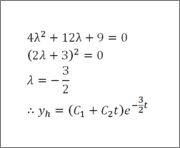# Mass-spring system: Steady state solution

yaro99

## Homework Statement

Find the steady-state motion of the mass–spring system modeled by the ODE:
4y''+12y'+9y=225-75sin(3t)

## Homework Equations

for a diff eq modeled as: my''+cy'+ky=F0cos(ωt),
yp=acos(ωt)+bsin(ωt)

a=F0*(m(ω022))/(m2*(ω022)22c2)

b=F0*(ωc)/(m2*(ω022)22c2)

## The Attempt at a Solution

I'm really not sure how to solve this since the equation given is not in the correct form (the right side is not in the form F0cos(ωt)).

Just to attempt the problem, I ignored the 225 and pretended that the right side was -75cos(3t)

m=4, c=12, k=9, F0=-75, ω=3, ω0=3/2

plugging in these values for a and b:
a=1, b=-4/3

which would make the (incorrect) solution: yp=cos(3t)-(4/3)sin(3t)

Is there another formula I should be using? The book has several other formulae listed for the chapter but doesn't explain them very well...

imiuru

## Homework Statement

Find the steady-state motion of the mass–spring system modeled by the ODE:
4y''+12y'+9y=225-75sin(3t)

The steady-state motion can be obtained by solving for homogenous solution of the ODE.Since the initial condition is not provided, you have C1 and C2 as constant in the solution.

imiuru
My last post was wrong as I mistook it as natural response of the system.

Well, in your case, you cannot ignore the "225" as it definitely will have effect on your spring system.

Basically, you should choose your yp as acos(ωt)+bsin(ωt) + c where c is the constant to take into account the 225

yaro99
My last post was wrong as I mistook it as natural response of the system.

Well, in your case, you cannot ignore the "225" as it definitely will have effect on your spring system.

Basically, you should choose your yp as acos(ωt)+bsin(ωt) + c where c is the constant to take into account the 225

If I try this method and solve by taking yp' and yp'' and plugging into the original equation, I am left with 3 unknowns and only 2 equations when I equate like terms.
Maybe I am equating the terms wrong. I had all the sin(3t) terms equal to -75, and all the other terms equal to 225.
I also tried making the sin(3t) equal to -75, the cos(3t) terms equal to 0, and the c term (which turned out to be 9c) equal to 225. I thought this to be the correct method; I got the correct value for c but not for the sin(3t) and cos(3t) terms.

Am I supposed to keep the a and b values I got in my original post and use those? If so, I still have the wrong sign for b, -4/3; the answer shows b to be +4/3.

imiuru
By equating all the coefficients of sin(3t) terms to -75, cos(3t) terms to 0, 9c to 225, you will be okay.

The answer provided is obviously wrong. There should be the minus sign.

yaro99
By equating all the coefficients of sin(3t) terms to -75, cos(3t) terms to 0, 9c to 225, you will be okay.

The answer provided is obviously wrong. There should be the minus sign.

Well, plugging it into Wolfram Alpha yields the book's answer, with the plus sign: http://www.wolframalpha.com/input/?i=4y''+12y'+9y=225-75sin(3t)

EDIT: I solved for yp again from scratch, without using my original values for a and b, and I did indeed get the correct answer, including the +4/3.
But I'm still not sure why the original equations I had for a and b gave me the wrong sign. Maybe it only applies if r(t)=F0cos(ωt) and no other constants or variables?

Last edited:
imiuru
Yeah. The answer should have +4/3.

Since there is no c in your original equations, you are now solving different set of equations, aren't you? If so, it's natural that you got something that's different from the correct answer, in this case wrong sign.

Last edited: